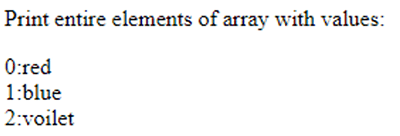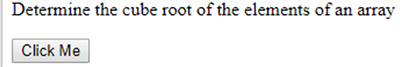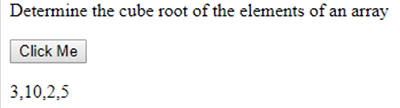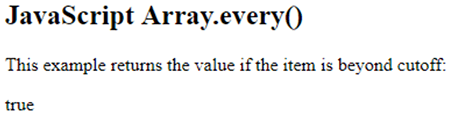Iteration Function in JavaScript || Learn JavaScript Online# Iteration Function in JavaScript

2157

Iteration functions in JavaScript are used to perform repetition in a code. There are several Array iteration methods that are available in JavaScript to write readable code. These methods permit iterations on each item present in an array.

Below are some Array iteration methods which have been found in JS.

forEach()

forEach() method executes the entire module once for each item existing in the array. This function permits the callback function to perform array mutation. However, this method does not allow any alternative to eliminate it.

Since forEach() is a user-defined function, developers can accomplish several operations on the existing array through it.

The syntax of forEach() is given below:

array.forEach(function callback(presentValue, index, array){

}[ThisArgmnt]);

The above-written syntax consists of three parameters i.e. presentValue, index and array. Among these parameters presentValue is mandatory whereas others are optional.

Program:

```<!DOCTYPE html>
<html>
<body>

<p>Print entire elements of array with values:</p>
<p id="demo"></p>

<script>
let bag = ["red", "blue", "voilet"];
bag.forEach(myFunc);

function myFunc(element, index1) {
document.getElementById("demo").innerHTML += index1 + ":" + element + "<br>";
}
</script>

</body>
</html>```array.map()

The JavaScript array.map() function is used to generate an array via the outcome of calling a provided function on each item in the array. When there is a need for applying a function on entire elements of an Array, the developer usually implements array.map() function.

The syntax is given below:

array.map(function(presentValue, index, array){

}[ThisArg]);

Let's illustrate array.map() through an example

```<!DOCTYPE html>
<html>
<body>

<p>Determine the cube root of the elements of an array</p>
<button onclick="myFunction()">Click Me</button>
<p id="demo"></p>

<script>
var numbers = [27, 1000, 8, 125];
function myFunction() {
x = document.getElementById("demo")
x.innerHTML = numbers.map(Math.cbrt );
}
</script>

</body>
</html>```

Output:On clicking on the "Click Me" button, the output appears as follow:.entries()

Object.entries() method is an iteration function which returns a novel array iterator object containing key/value pairs associated with each item of Array.

Syntax:

array.entries()

Program:

```var bucket = ['red', 'blue', 'green'];
var iterator2 = bucket.entries();
console.log(iterator2.next().value);
console.log(iterator2.next().value);
```

Output:

> Array [0, "red"]

> Array [1, "blue"]

.every()

Array.every() is the most remarkable function used in JS to verify whether entire items present in an array fulfill the provided condition or not. This function returns a Boolean value i.e. true or false. If the condition is satisfied, it returns true else it returns false.

Following is the syntax of .every()

`array5.every(function(presentValue, index, ar), thisValue)`

Program:

```<!DOCTYPE html>
<html>
<body>

<h2>JavaScript Array.every()</h2>
<p>This example returns the value if the item is beyond cutoff:</p>
<p id="demo"></p>

<script>
// JavaScript code for every() function
function cutoff(item, index, array)
{
return item > 11;
}

function func() {
var arr = [ 23, 78, 79, 95, 27 ];
// check for positive
// number
var value1 = arr.every(cutoff);
document.write(value1);
}
func();
</script>

</body>
</html>```

Output:The reduceRight() method:

The reduceRight() method is used in JavaScript in order to perform the reduction of an array. In this, a function is simultaneously being applied across values to reduce it to a single value.

However, the callback function is executed at once for an entire array.

```<!DOCTYPE html>
<html>
<body>

<h2>JavaScript Array.reduceRight()</h2>
<p>This program find the addition of all items in an array:</p>
<p id="demo"></p>

<script>
var numbers = [6, 4, 8, 1, 5];

function myFunction(add, value1, index, array) {
}
</script>

</body>
</html>```

Conclusion:

These were the major iteration methods that are generally used in JavaScript. Correspondingly, there are many other in-built iterator functions such as Array filter(), Array indexOf() etc.Share:## Get a .COM for just \$6.98

Secure Domain for a Mini Price